# VBA Sum Function (Ranges, Columns, & More)

This tutorial will show you how to use the Excel Sum function in VBA

The sum function is one of the most widely used Excel functions, and probably the first one that Excel users learn to use. VBA does not actually have an equivalent – a user has to use the built-in Excel function in VBA using the WorkSheetFunction object.

## Sum WorksheetFunction

The WorksheetFunction object can be used to call most of the Excel functions that are available within the Insert Function dialog box in Excel. The SUM function is one of them.

``````Sub TestFunction
Range("D33") = Application.WorksheetFunction.Sum("D1:D32")
End Sub``````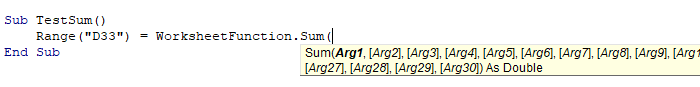You are able to have up to 30 arguments in the SUM function. Each of the arguments can also refer to a range of cells.

This example below will add up cells D1 to D9

``````Sub TestSum()
Range("D10") = Application.WorksheetFunction.SUM("D1:D9")
End Sub``````

The example below will add up a range in column D and a range in column F. If you do not type the Application object, it will be assumed.

``````Sub TestSum()
Range("D25") = WorksheetFunction.SUM (Range("D1:D24"), Range("F1:F24"))
End Sub``````

Notice for a single range of cells you do not have to specify the word ‘Range’ in the formula in front of the cells,  it is assumed by the code. However, if you are using multiple arguments, you do need to do so.

## Assigning a Sum result to a Variable

You may want to use the result of your formula elsewhere in code rather than writing it directly back to and Excel Range. If this is the case, you can assign the result to a variable to use later in your code.

``````Sub AssignSumVariable()
Dim result as Double
'Assign the variable
result = WorksheetFunction.SUM(Range("G2:G7"), Range("H2:H7"))
'Show the result
MsgBox "The total of the ranges is " &  result
End Sub``````

## Sum a Range Object

You can assign a group of cells to the Range object, and then use that Range object with the WorksheetFunction object.

``````Sub TestSumRange()
Dim rng As Range
'assign the range of cells
Set rng = Range("D2:E10")
'use the range in the  formula
Range("E11") = WorksheetFunction.SUM(rng)
'release the range object
Set rng = Nothing
End Sub``````

## Sum Multiple Range Objects

Similarly, you can sum multiple Range Objects.

``````Sub TestSumMultipleRanges()
Dim rngA As Range
Dim rngB as Range
'assign the range of cells
Set rngA = Range("D2:D10")
Set rngB = Range("E2:E10")
'use the range in the formula
Range("E11") = WorksheetFunction.SUM(rngA, rngB)
'release the range object
Set rngA = Nothing
Set rngB = Nothing
End Sub``````

## Sum Entire Column or Row

You can also use the Sum function to add up an entire column or an entire row

This procedure below will add up all the numeric cells in column D.

``````Sub TestSum()
Range("F1") = WorksheetFunction.SUM(Range("D:D")
End Sub``````

While this procedure below will add up all the numeric cells in Row 9.

``````Sub TestSum()
Range("F2") = WorksheetFunction.SUM(Range("9:9")
End Sub``````

## VBA Coding Made Easy

Stop searching for VBA code online. Learn more about AutoMacro - A VBA Code Builder that allows beginners to code procedures from scratch with minimal coding knowledge and with many time-saving features for all users!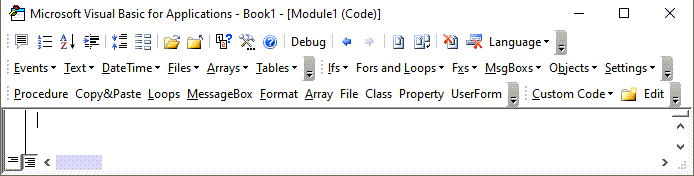## Sum an Array

You can also use the WorksheetFunction.Sum to add up values in an array.

``````Sub TestArray()
Dim intA(1 To 5) As Integer
Dim SumArray As Integer
'populate the array
intA(1) = 15
intA(2) = 20
intA(3) = 25
intA(4) = 30
intA(5) = 40
'add up the array and show the result
MsgBox WorksheetFunction.SUM(intA)
End Sub``````

## Using the SumIf Function

Another worksheet function that can be used is the SUMIF function.

``````Sub TestSumIf()
Range("D11") = WorksheetFunction.SUMIF(Range("C2:C10"), 150, Range("D2:D10"))
End Sub``````

The procedure above will only add up the cells in Range(D2:D10) if the corresponding cell in column C = 150.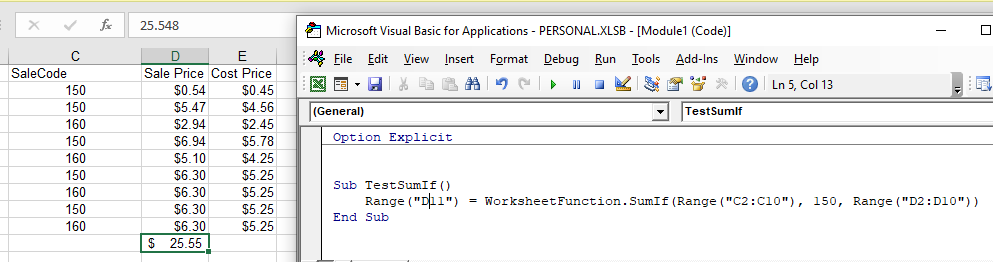## Sum Formula

When you use the WorksheetFunction.SUM to add a sum to a range in your worksheet, a static sum is returned, not a flexible formula. This means that when your figures in Excel change, the value that has been returned by the WorksheetFunction will not change.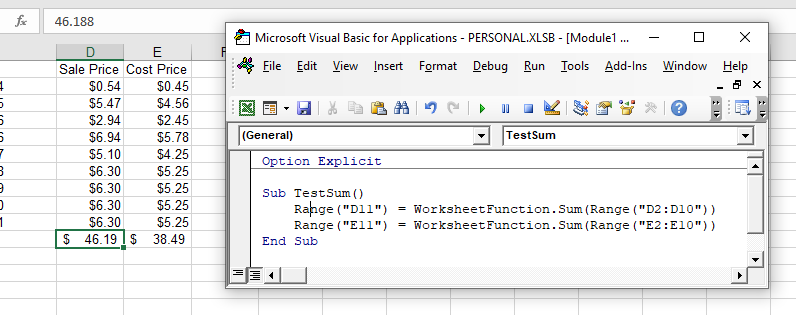In the example above, the procedure TestSum has added up Range(D2:D10) and the result has been put in D11. As you can see in the formula bar, this result is a figure and not a formula.

If any of the values change therefore in the Range(D2:D10), the result in D11 will NOT change.

Instead of using the WorksheetFunction.SUM, you can use VBA to apply a Sum Function to a cell using the Formula or FormulaR1C1 methods.

VBA Programming | Code Generator does work for you!

### Formula Method

The formula method allows you to point specifically to a range of cells eg: D2:D10 as shown below.

``````Sub TestSumFormula
Range("D11").Formula = "=SUM(D2:D10)"
End Sub``````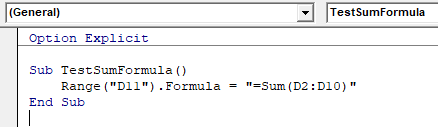### FormulaR1C1 Method

The FromulaR1C1 method is more flexible in that it does not restrict you to a set range of cells. The example below will give us the same answer as the one above.

``````Sub TestSumFormula()
Range("D11").FormulaR1C1 = "=SUM(R[-9]C:R[-1]C)"
End Sub``````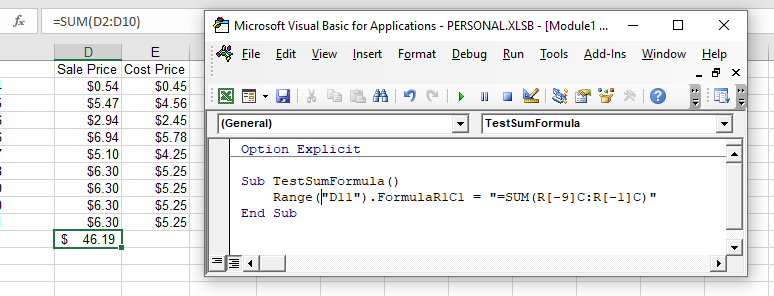However, to make the formula more flexible, we could amend the code to look like this:

``````Sub TestSumFormula()
ActiveCell.FormulaR1C1 = "=SUM(R[-9]C:R[-1]C)"
End Sub``````

Wherever you are in your worksheet, the formula will then add up the 8 cells directly above it and place the answer into your ActiveCell. The Range inside the SUM function has to be referred to using the Row (R) and Column (C) syntax.

Both these methods enable you to use Dynamic Excel formulas within VBA.

There will now be a formula in D11 instead of a value.